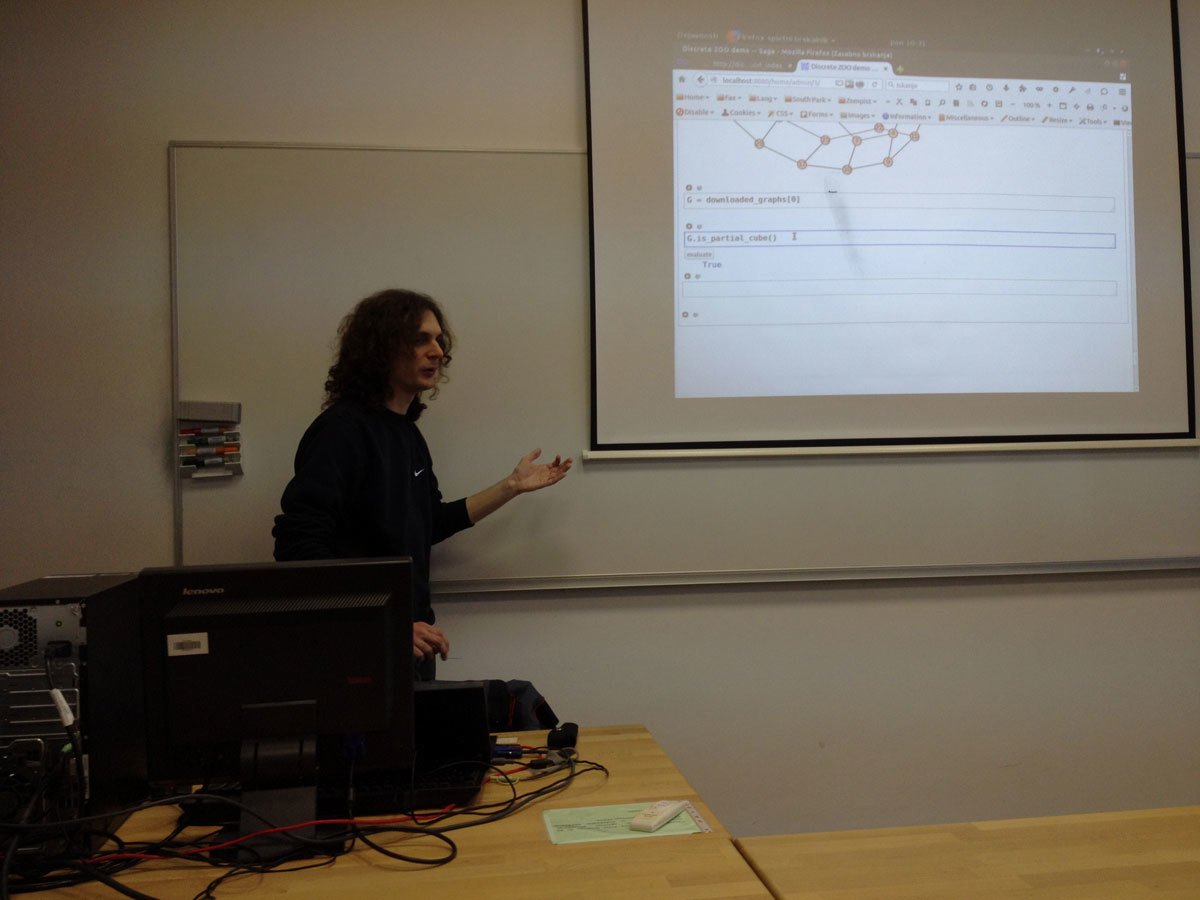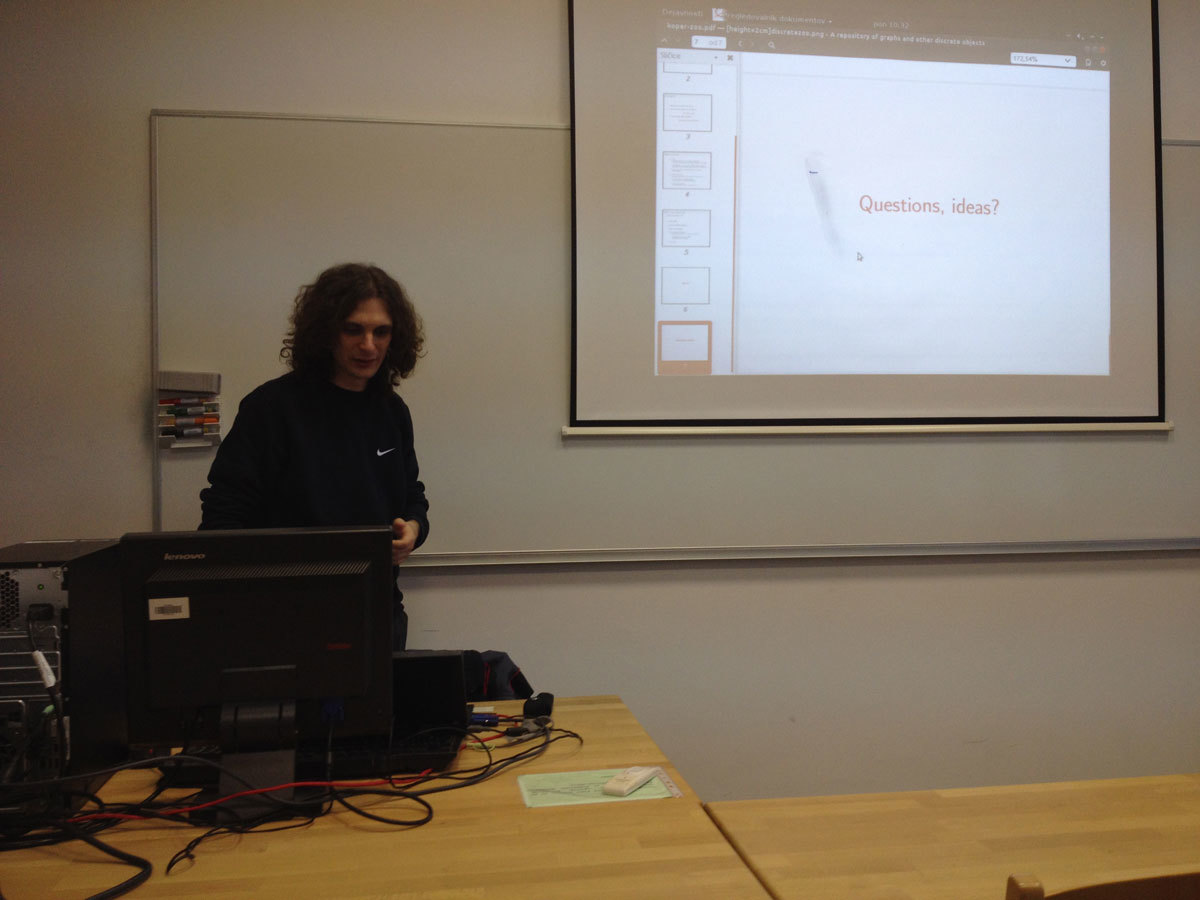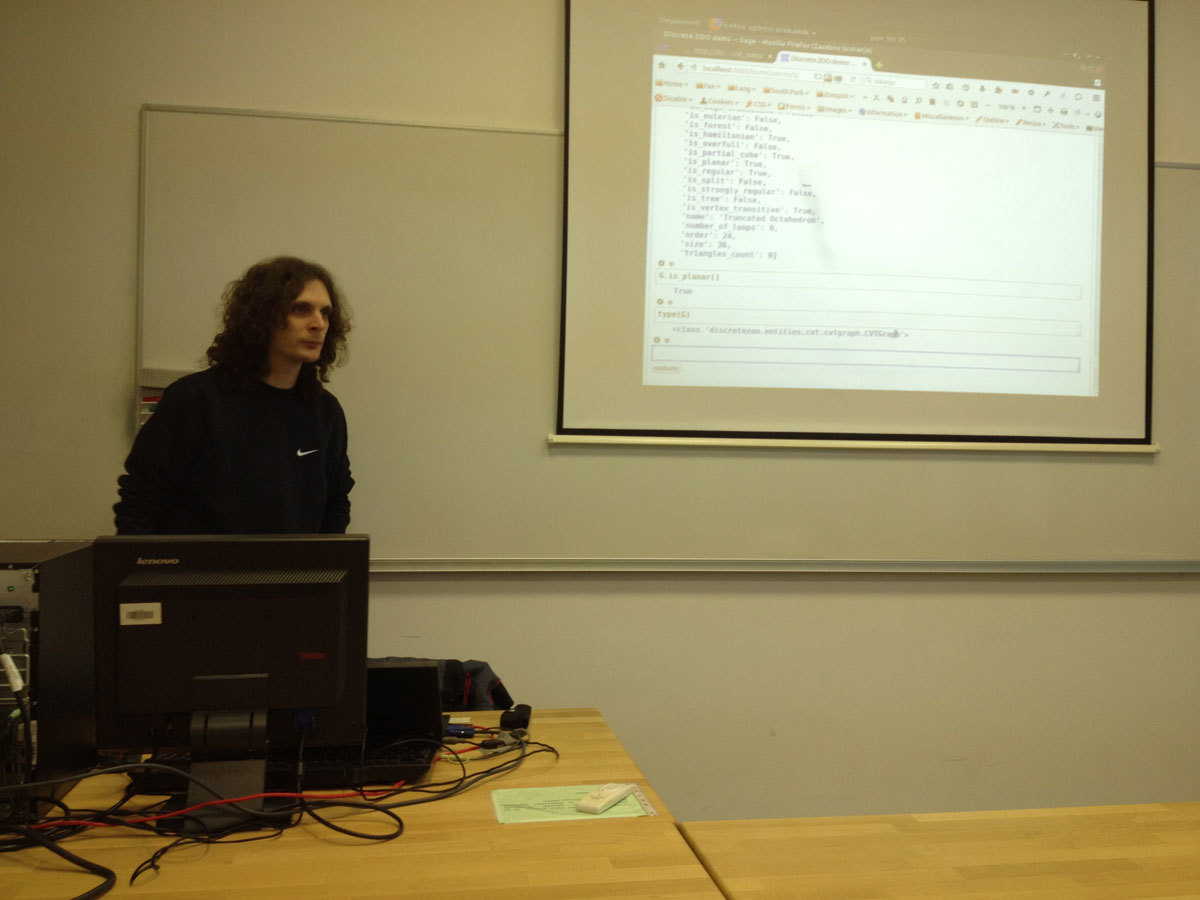Univerza na Primorskem Fakulteta za matematiko, naravoslovje in informacijske tehnologije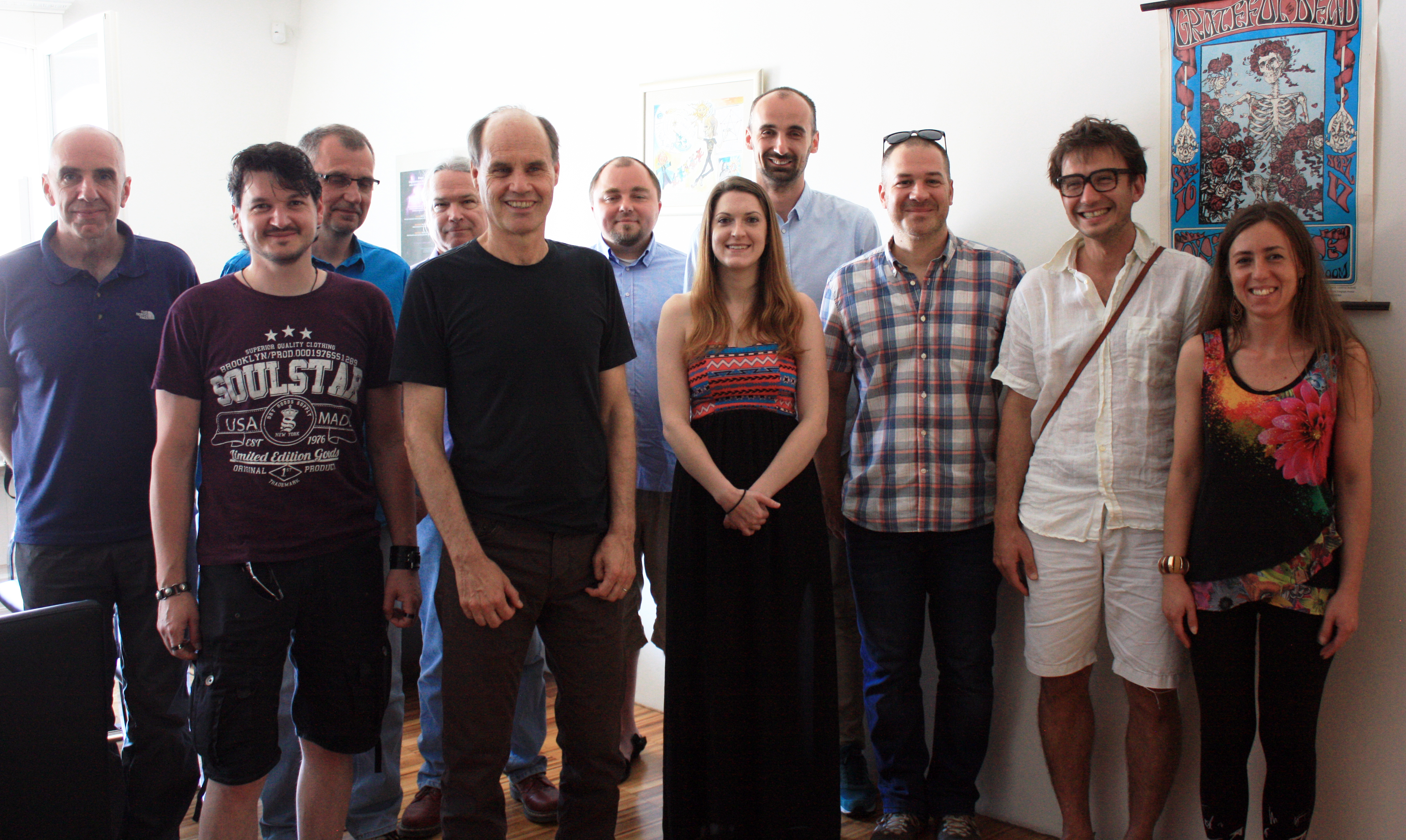SI | EN# Raziskovalni matematični seminar - Arhiv

 2023 2022 2021 2020 2019 2018 2017 2016 2015 2014 2013 2012 2011 2010
 1 2 3 4 5 6 7 8 9 10 11 12
Datum in ura / Date and time: 29.2.16
(10:00-11:00)
Predavalnica / Location: FAMNIT-POŠTA
Predavatelj / Lecturer: Safet Penjić (UP IAM)
Naslov / Title: On the Terwilliger algebra of bipartite distance-regular graphs with \Delta_2=0 and c_2=2
Vsebina / Abstract:

Let \Gamma denote a bipartite distance-regular graph with diameter D \ge 4 and valency k \ge 4. Let X denote the vertex set of \Gamma, and let A denote the adjacency matrix of \Gamma. For x \in X and for 0 \le i \le D, let \G_i(x) denote the set of vertices in X that are distance i from vertex x. Define a parameter \Delta_2 in terms of the intersection numbers by \Delta_2 = (k-2)(c_3-1)-(c_2-1)p^2_{22}. It is known that \Delta_2 = 0 implies that D \le 5 or c_2 \in \{1,2\}.

For x \in X let T=T(x) denote the subalgebra of \Mat_X(\C) generated by A, \Es_0, \Es_1, ..., \Es_D, where for 0 \le i \le D, \Es_i represents the projection onto the i-th subconstituent of \Gamma with respect to x. We refer to T as the Terwilliger algebra of \Gamma with respect to x. By the endpoint of an irreducible T-module W we mean min\{i | \Es_iW \ne 0\}.

Building on the work of [MacLean, Miklavič and Penjić: On the Terwilliger algebra of bipartite distance-regular graphs with \Delta_2=0 and c_2=1, Linear Algebra and its Applications 496 (2016)] we find the structure of nonthin irreducible T-modules of endpoint 2 for graphs \Gamma in which for 2\le i\le D-1 there exist complex scalars \alpha_i, \beta_i with the following property: for all x, y, z \in X such that \partial(x, y) = 2, \partial(x, z) = i,  \partial(y, z) = i we have \alpha_i + \beta_i  |\G_1(x) \cap \G_1(y) \cap \G_{i-1}(z)| = |\G_{i-1}(x) \cap \G_{i-1}(y) \cap \G_1(z)|; in case when \Delta_2=0 and c_2=2.

We show that if \Gamma is not almost 2-homogeneous, then up to isomorphism there exists exactly one irreducible T-module with endpoint 2 and it is not thin. We give an basis for this T-module, and we give the action of A on this basis. The isomorphism class of such a given module is determined by the intersection numbers of the \Gamma.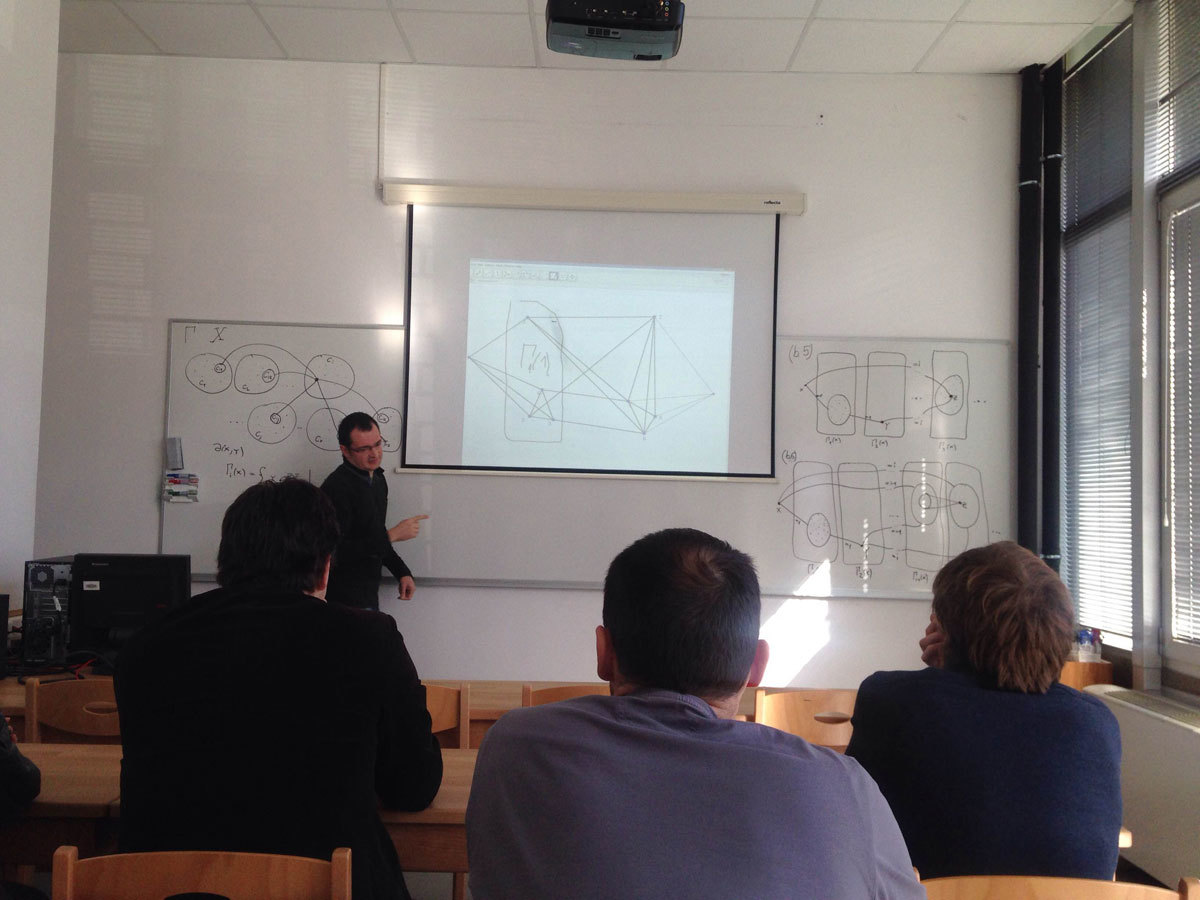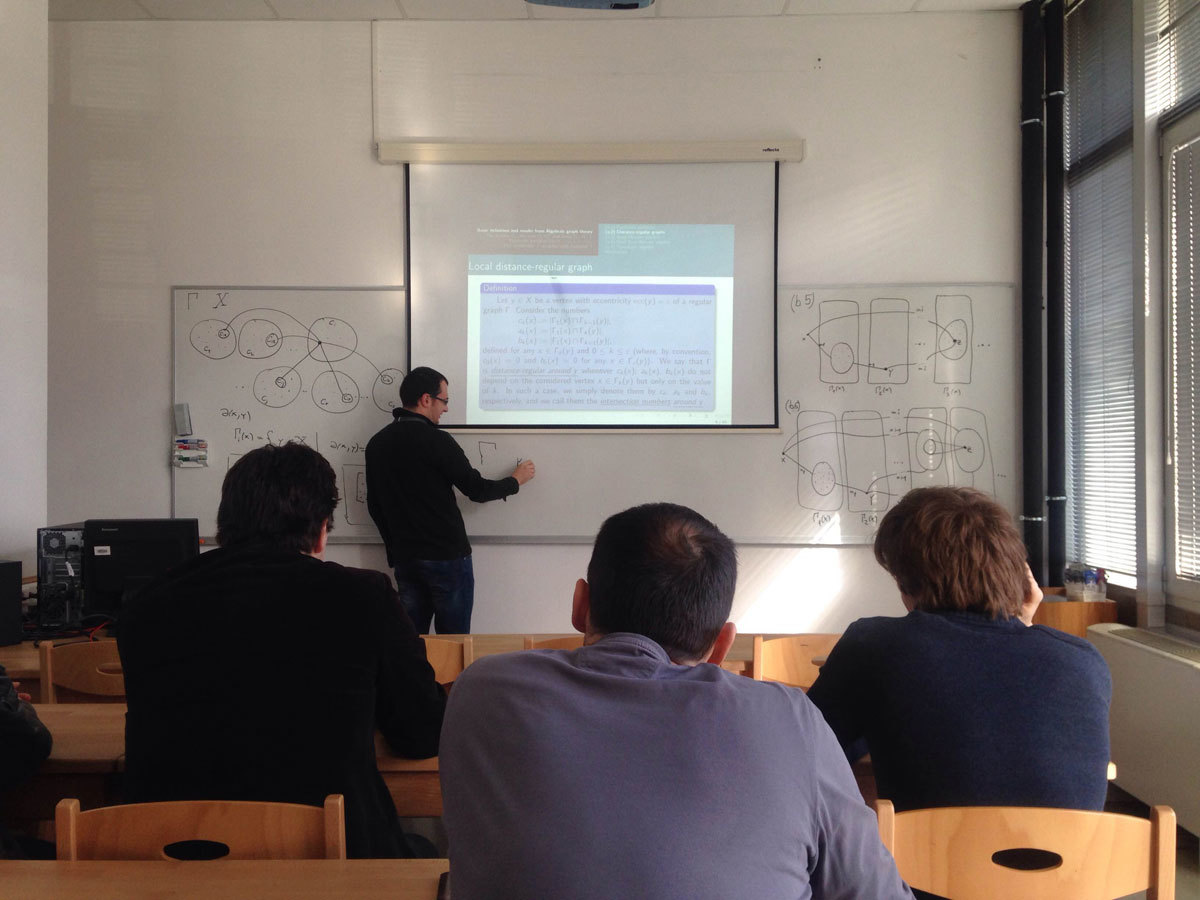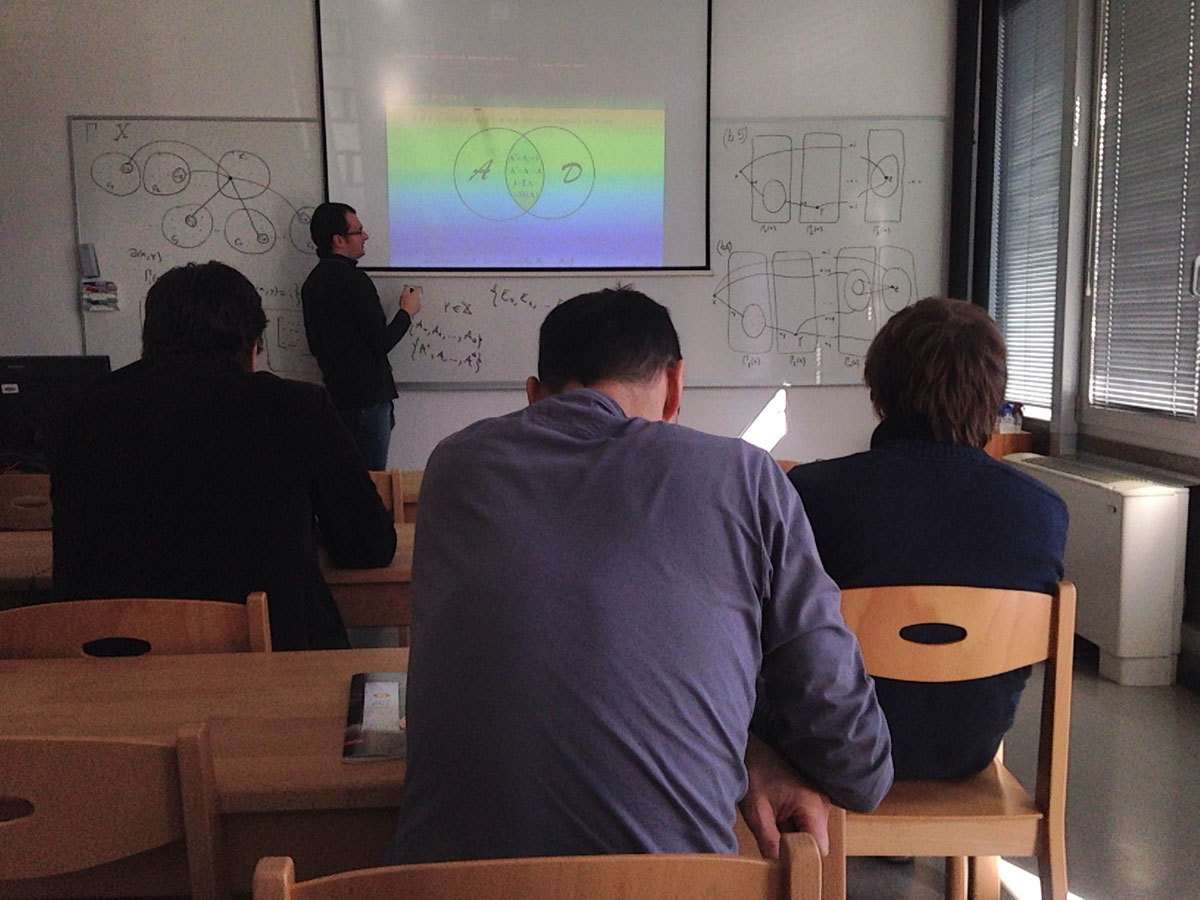Datum in ura / Date and time: 22.2.16
(10:00-11:00)
Predavalnica / Location: FAMNIT-POŠTA
Predavatelj / Lecturer: Janoš Vidali (University of Ljubljana)
Naslov / Title: DiscreteZOO - a repository of graphs and its companion Sage package
Vsebina / Abstract:

DiscreteZOO is a project combining a central repository of graphs, its website front-end and extensions for software packages like Sage. The repository contains certain precomputed properties to speed up the processes of filtering, searching and computation. For now it can store graphs, with the groundwork already laid out for more combinatorial objects (such as maps, maniplexes, geometries, etc.).

In the talk we will show how one can interact with DiscreteZOO on the example of the censuses of vertex transitive graphs by Royle, Conder, and Potočnik, Spiga and Verret. We will perform some example searches on the website, download a subset of the database and showcase some queries that can be run locally with the Sage package.